# Model And Control A Manipulator Arm With Robotics And Simscape

Execute a pick-and-place workflow using an ABB YuMi robot, which demonstrates how to design robot algorithms in Simulink®, and then simulate the action in a test environment using Simscape™. The example also shows how to model a system with different levels of fidelity to focus on better focus on the associated algorithm design.

The design elements of this example are split into three sections to more easily focus on different aspects of the model design:

1. Create a Task & Trajectory Scheduler for Pick-And-Place using Simplified Manipulator System Dynamics:

2. Add Core Manipulator Dynamics and Design a Controller

3. Verify Complete Workflow on Simscape Model of the Robot and Environment

### High Level Goals

In the Interactively Build a Trajectory For an ABB YuMi Robot example, a robot waypoint sequence was designed and replayed using a continuous trajectory. In this example, Simulink models convert these waypoints to a complete and repeatable pick-and-place workflow. The model has the two key elements:The task scheduling and trajectory generation portion defines how the robot traverses through the states. This includes the robot configuration state at any instant, what the goal position is, whether the gripper should be open or closed, and the current trajectory being sent to the robot.

The system dynamics portion models the robot behavior. This defines how the robot moves given a set of reference trajectories and a boolean gripper command (open or closed). The system dynamics can be modeled with different levels of fidelity, depending on the aim of the overall model.

For this example, during the task scheduler design, the aim is to ensure the scheduler behaves correctly under the assumption that the robot is under stable motion control. For this portion, a straightforward model that simulates quickly desirable, so the system dynamics are modeld using the Joint Space Motion Model block. This block simulates the manipulator motion given joint-space reference trajectories under a stable controller with predefined response parameters. Once the task scheduling is complete, the focus of the model is shifted to controller design and system verification, which requires more complex system dynamics models.

### Define a Robot and Environment

Load an ABB YuMi robot model. The robot is an industrial manipulator with two arms. This example only uses a single arm.

`robot = loadrobot('abbYumi','Gravity',[0 0 -9.81]);`

Create a visualization to replay simulated trajectories.

```iviz = interactiveRigidBodyTree(robot); ax = gca;```

Add an environment by creating a set of collision objects using an example helper function.

`exampleHelperSetupWorkspace(ax);`### Initialize Shared Simulation Parameters

This example uses a set of predefined configurations, `configSequence`, as robot states. These are stored in an associated MAT file and were initially defined in Interactively Build a Trajectory For an ABB YuMi Robot.

`load abbSavedConfigs.mat configSequence`

For the simulation, the initial state of the robot must be defined including postion, velocity, and acceleration of each joint.

```% Define initial state q0 = configSequence(:,1); % Position dq0 = zeros(size(q0)); % Velocity ddq0 = zeros(size(q0)); % Acceleration```

### Create a Task & Trajectory Scheduler

Load the first model, which focuses on the task scheduling and trajectory generation section of the model.

`open_system('modelWithSimplifiedSystemDynamics.slx');`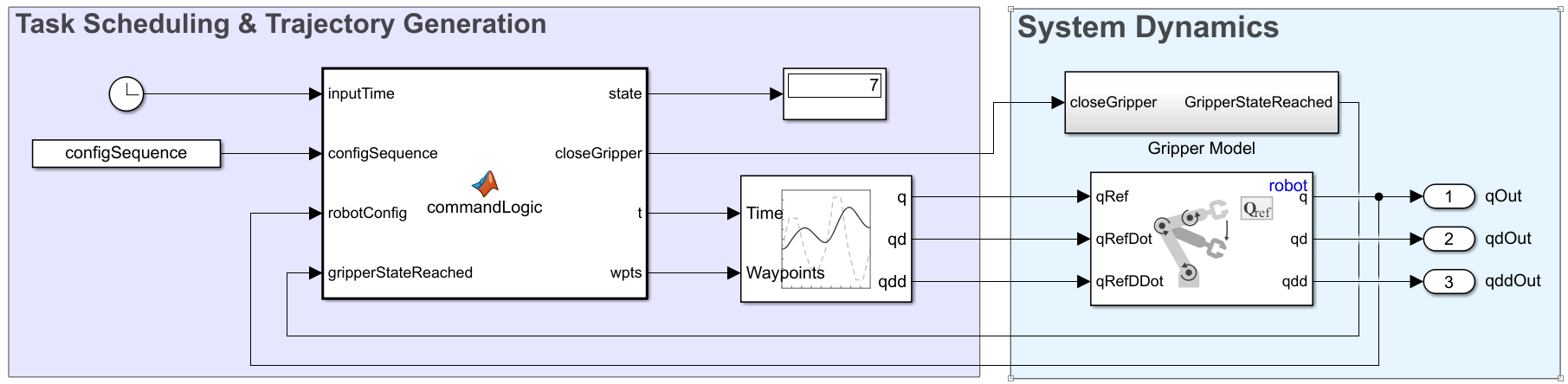#### Simplified System Dynamics

To focus on the scheduling portion of the model, the system dynamics are modeled using the Joint Space Motion Model block. This motion model assumes the robot can reach specified configurations under stable, accurate control. Later, the example details more accurate modelling of the system dynamics.

The gripper is modeled as a simple Boolean command input as `0` or `1` (open or closed), and an output that indicates whether the gripper achieved the commanded position. Typically, robots treat the gripper command separately from the other configuration inputs.

The series of tasks the robot through are eight states:The scheduler is implemented using a MATLAB Function block, `commandLogic`. The scheduler advances states when the gripper state is reached and all the manipulator joints have reached their target positions within a predefined threshold. Each task is input to the Trapezoidal Velocity Profile Trajectory block which generates a smooth trajectory between each waypoint.

#### Simulate the Model

The provided Simulink model stores variables relevant to the example in the Model Workspace. Click Load Default Parameters to reinitialize the variables if needed. For more information, see Model Workspaces (Simulink).

Run the model by calling `sim`.

Use the interactive visualization to play back the motion. The model is simulated for a few extra seconds to ensure that the cycle loops as expected after the first motion. This model does not simulate any environment interaction, so the robot does not actually grab objects in this simulation.

```simout = sim('modelWithSimplifiedSystemDynamics.slx'); % Visualize the motion using the interactiveRigidBodyTree object. iviz.ShowMarker = false; iviz.showFigure; rateCtrlObj = rateControl(length(simout.tout)/(max(simout.tout))); for i = 1:length(simout.tout) iviz.Configuration = simout.yout{1}.Values.Data(i,:); waitfor(rateCtrlObj); end```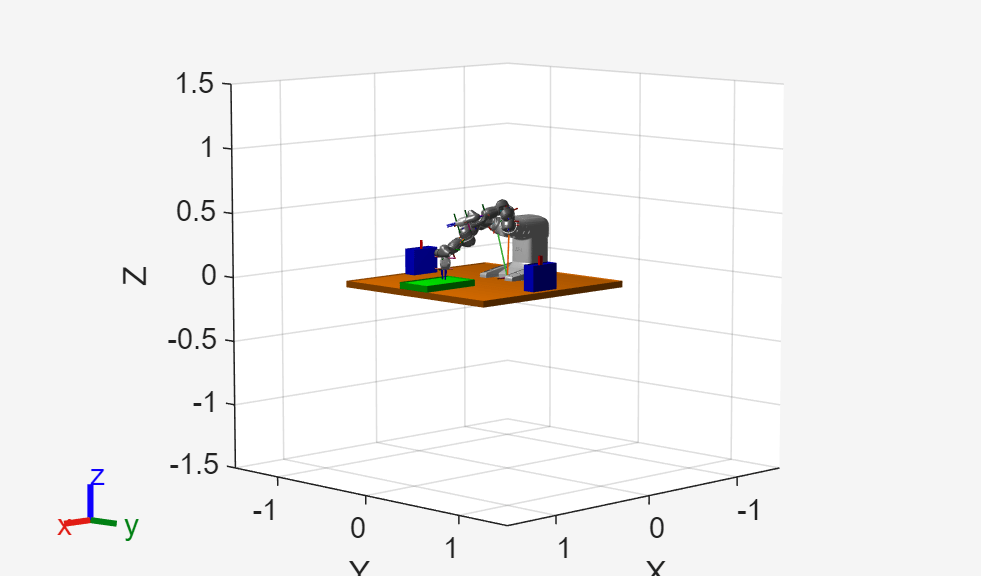### Add Core Manipulator Dynamics and Design a Controller

Now that the scheduler has been designed and verified, add a controller for the robot with two elements

• A more complex manipulator dynamics model that accepts joint torques and gripper commands

• A joint-space controller that returns joint torques given desired and current manipulator states

Open the next provided model with the added controller.

`open_system('modelWithControllerAndBasicRobotDynamics.slx');`#### Manipulator Dynamics

For the purpose of designing a controller, the manipulator dynamics have to represent the manipulator joint positions given torque inputs. This is achieved inside the Manipulator Dynamics subsystem using a Forward Dynamics block to convert joint torques to joint acceleration given the current state, and then integrating twice to get the complete joint configuration. The integrators are initialized to `q0` and `dq0`, the initial joint position and velocity.Additionally, the gripper control subsystem overrides the joint control torques to the gripper actuators with 10 N of force that is applied to close or open the gripper.

Note that the second integrator is saturated.While manipulators under well-designed position controllers typically will not reach joint limits, the addition of open-loop forces from the gripper action mean that joint limits are required to ensure a realistic response. For a more accurate model, the joint saturation could be connected to the velocity to reset integration, but for this model, this level of accuracy is sufficient.

#### Gripper Sensor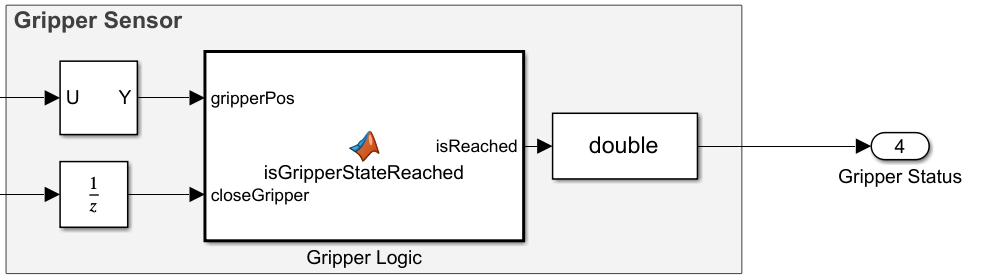This model also adds a more detailed gripper sensor to check when the gripper has actually been opened or closed. The gripper sensor extracts the last two values of the joint configuration (the values that correspond to the gripper position), and compares them to the intended gripper position, given by the `closeGripper` command, in a MATLAB Function block, Gripper Logic. The Gripper Status returns 1 when the positions of the gripper joints match the desired state given by the closeGripper command. When the gripper has not yet reached those states, Gripper Status returns zero. This matches the behavior of the Gripper Model in the earlier, simplified model.

#### Joint-Space Controller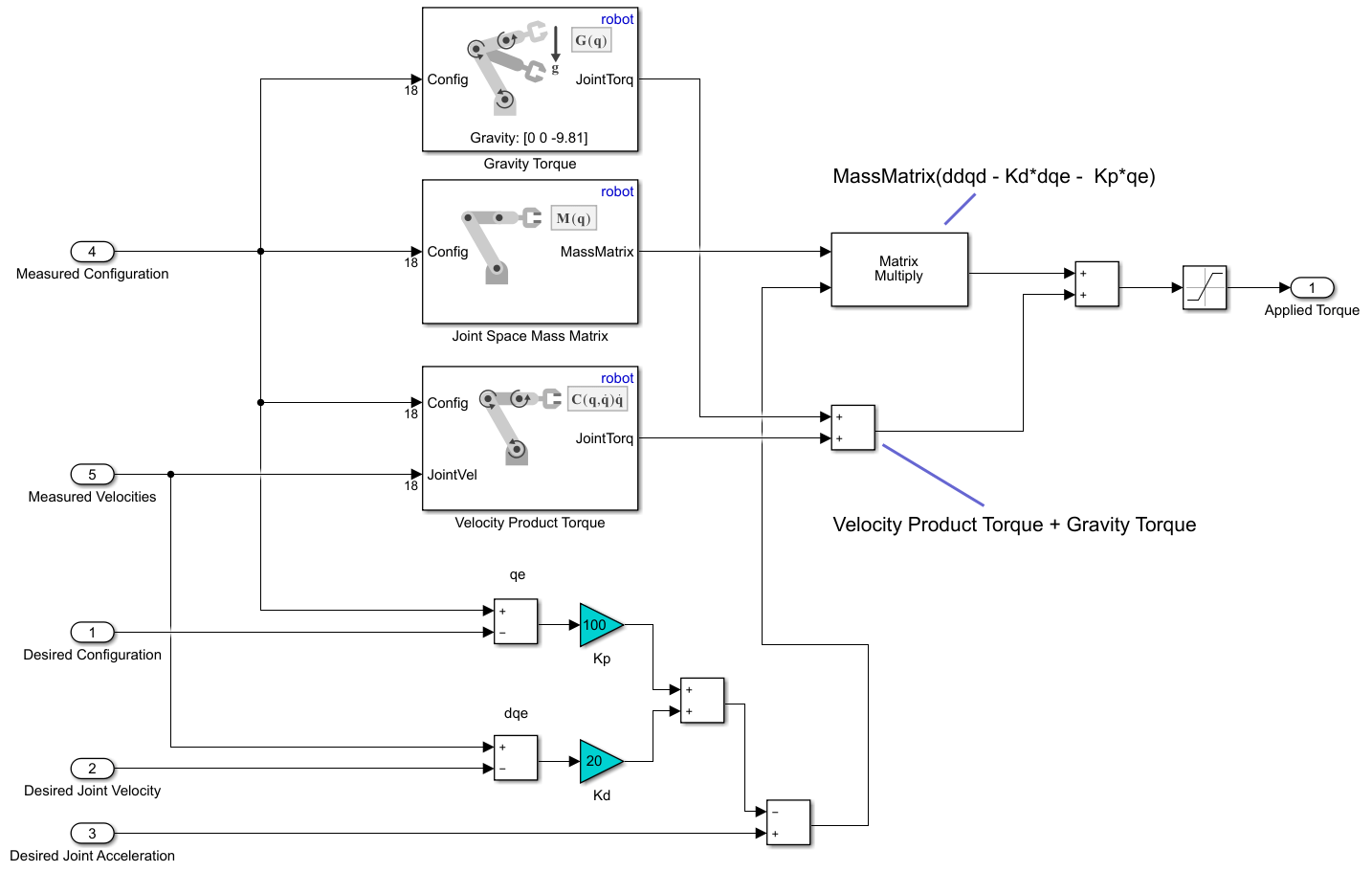This model also adds a computed torque controller which implements a model-based approach to joint control. For more details, see Build Computed Torque Controller Using Robotics Manipulator Blocks in the Perform Safe Trajectory Tracking Control Using Robotics Manipulator Blocks example. This model uses the same controller, but with the ABB YuMi as the `rigidBodyTree` input rather than the Rethink Sawyer.

#### Simulate the Model

Simulate and visualize the results using the new model.

```simout = sim('modelWithControllerAndBasicRobotDynamics.slx'); % Visualize the motion using the interactiveRigidBodyTree iviz.ShowMarker = false; iviz.showFigure; rateCtrlObj = rateControl(length(simout.tout)/(max(simout.tout))); for i = 1:length(simout.tout) iviz.Configuration = simout.yout{1}.Values.Data(i,:); waitfor(rateCtrlObj); end```### Verify Complete Workflow on Simscape Model of the Robot and Environment

Now that the task scheduler and controller have been designed, add more complex robot and environment models. Use Simscape Multibody™ which can create high-fidelity models of physical systems. In this application, Simscape adds dynamics with built-in joint limits and contact modeling. This last step adds simulation accuracy at the cost of modeling complexity and simulation speed. Simcape also provides a built-in visualization, Mechanics Explorer, that can be viewed during and after simulation.

Load the final provided model, which has the same top view.

`open_system('modelWithSimscapeRobotAndEnvironmentDynamics.slx');`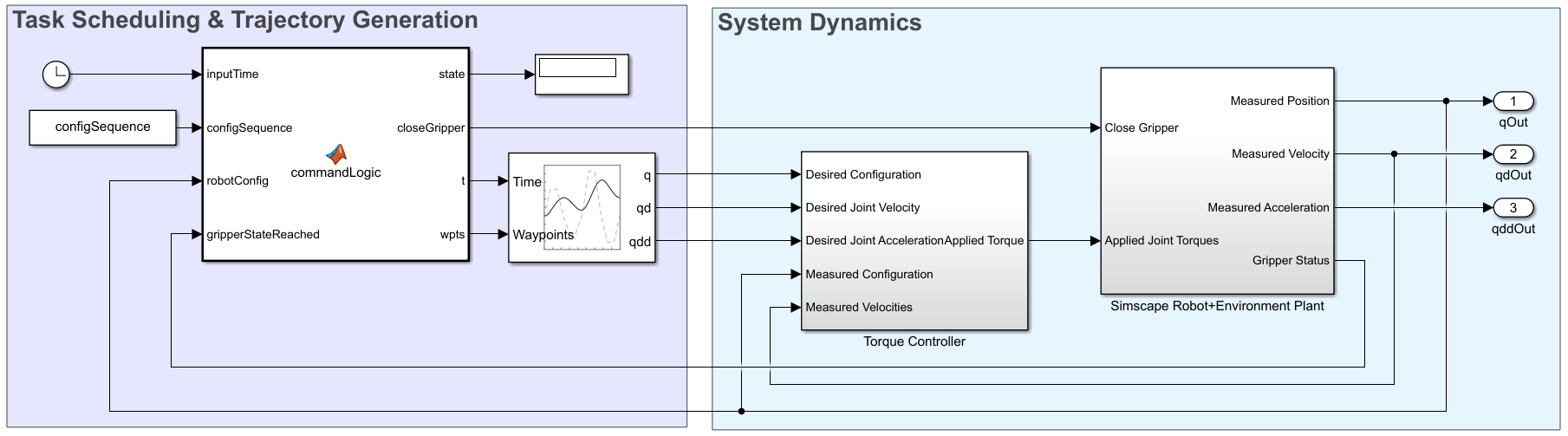### Simscape Robot & Environment Plant

This main different from the previous model is the plant model. The core manipulator dynamics from the previous model have been replaced with a Simscape model for the robot and the environment: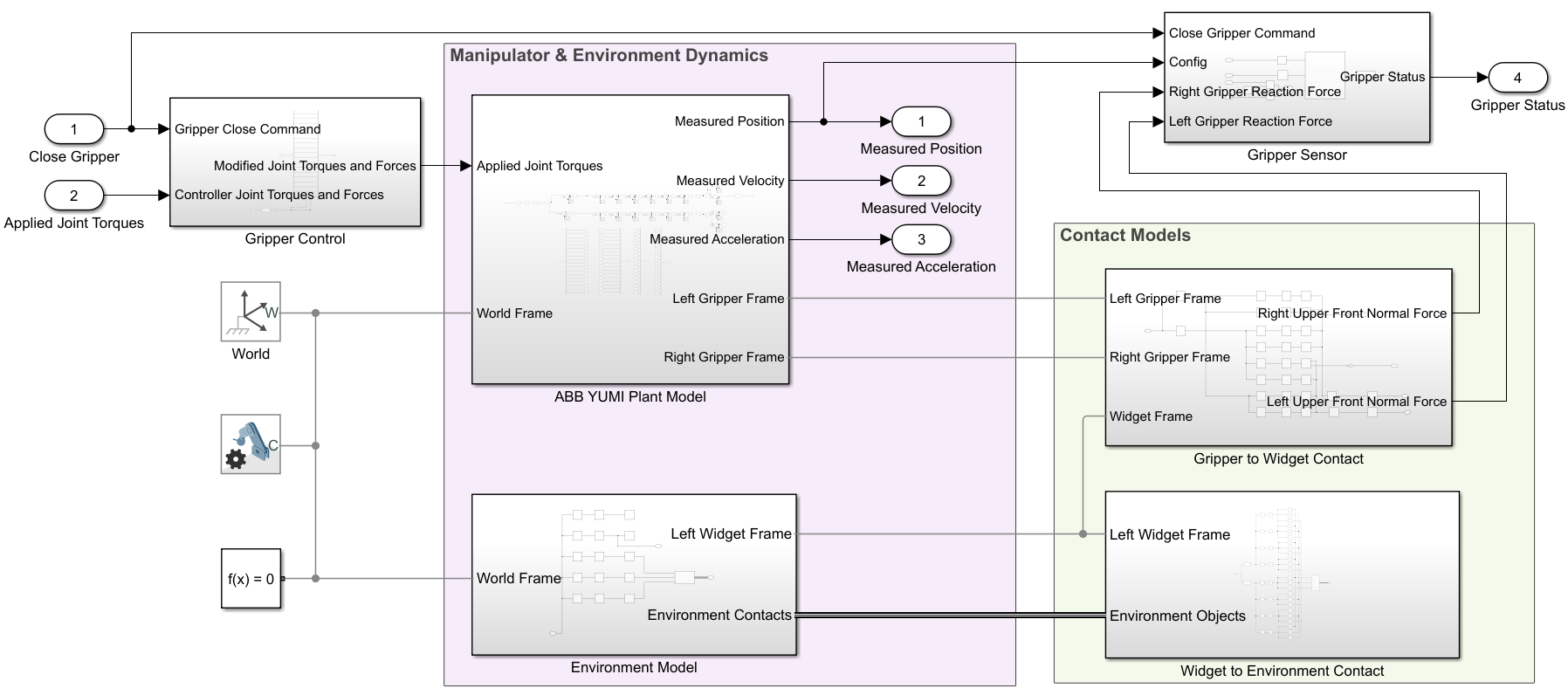#### Manipulator & Environment Dynamics

The manipulator and environment are constructed using Simscape Multibody. The robot model was created by calling `smimport` on the robot URDF file with the provided meshes. Then, the joints were actuated with joint torques by linking via muxes and GoTo tags and outfitted with sensors that return joint position, velocity, and acceleration.

The objects,or widgets, are actually picked up in this simulation, so define the widget size.

`widgetDimensions = [0.02 0.02 0.07];`

#### Contact Models

The contact in this model is split into two categories:

• Contact between the gripper and the widget

• Contact between the widget and the environment

In both cases, contact proxies are used in lieu of direct surface-to-surface contact. The use of contact proxies speed up modeling to improve performance. For the gripper-widget contact, the gripper contacts are modeled using two brick solids, while eight spherical contacts are used to model the widget interface. Similarly, the widget-to-environment contact uses spheres at each of the four corners of the widget that contact the brick solids representing the environment.

Define the parameters for the contact models to be close to their default states.

```% Contact parameters stiffness = 1e4; damping = 30; transition_region_width = 1e-4; static_friction_coef = 1; kinetic_friction_coef = 1; critical_velocity = 1;```

#### Gripper Control & Sensing

The Gripper Control is the same, but the Gripper Sensor is modified. Since this gripper can actually pick up objects, the closed gripper state is reached when the grasp is firm. The actual closed position may never be reached. Therefore, extra logic has been added that returns a value, `isGrippingObj`, that is true when both the left and right gripper reaction forces exceed a threshold value. The Gripper Logic MATLAB Function block accepts this variable as an input.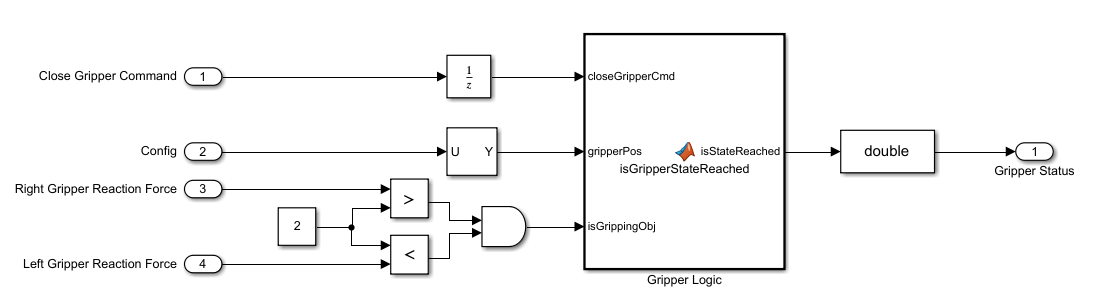#### Simulate the Model

Simulate the robot. Due to the high complexity, this may take several minutes.

`simout = sim('modelWithSimscapeRobotAndEnvironmentDynamics.slx');`

Use the Mechanics Explorer to visualize the performance during and after simulation.#### Extensibility

This example has focused on the design of a scheduling and control system for a pick-and-place application. Further investigations might include the effect of sampling on the controller, the impact of unexpected contact using Simscape Multibody, or the extension to a multi-domain model like detailing the behavior of the electrical motors that the robot uses.